Courses

# Chapter 4 - Pointers , Chapter Notes, Class 12, Computer Science | EduRev Notes

## COMPUTER SCIENCE for Class 12(XII) - CBSE & NCERT Curriculum

Created by: Imk Pathsala

## Class 12 : Chapter 4 - Pointers , Chapter Notes, Class 12, Computer Science | EduRev Notes

The document Chapter 4 - Pointers , Chapter Notes, Class 12, Computer Science | EduRev Notes is a part of the Class 12 Course COMPUTER SCIENCE for Class 12(XII) - CBSE & NCERT Curriculum.
All you need of Class 12 at this link: Class 12

Chapter 4 - Pointers , Chapter Notes, Class 12,  Computer Science

POINTERS

- Pointer is a variable that holds a memory address of another variable of same type.
- It supports dynamic allocation routines.
- It can improve the efficiency of certain routines.

C++MemoryMap :
- Program Code : It holds the compiled code of the program.
- Global Variables : They remain in the memory as long as program continues.
- Stack : It is used for holding return addresses at function calls, arguments passed to
the functions, local variables for functions. It also stores the current state of the CPU.
- Heap : It is a region of free memory from which chunks of memory are allocated via
DMA functions.

StaticMemory Allocation : The amount of memory to be allocated is known in advance and
it allocated during compilation, it is referred to as Static Memory Allocation.
e.g. int a; // This will allocate 2 bytes for a during compilation.

Dynamic Memory Allocation : The amount of memory to be allocated is not known
beforehand rather it is required to allocated as and when required during runtime, it is
referred to as dynamic memory allocation.
C++ offers two operator for DMA – new and delete.
e.g int x =new int; float y= new float; // dynamic allocation
delete x; delete y; //dynamic deallocation

Free Store : It is a pool of unallocated heap memory given to a program that is used by the
program for dynamic memory allocation during execution.
Declaration and Initialization of Pointers :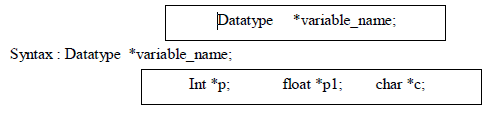Eg. Int *p; float *p1; char *c;
Two special unary operator * and & are used with pointers. The & is a unary operator that
returns the memory address of its operand.
Eg. Int a = 10; int *p; p = &a;

Pointer arithmetic:
Two arithmetic operations, addition and subtraction, may be performed on pointers.
When you add 1 to a pointer, you are actually adding the size of whatever the pointer is
pointing at. That is, each time a pointer is incremented by 1, it points to the memory location
of the next element of its base type.
e.g. int *p; P++;
If current address of p is 1000, then p++ statement will increase p to 1002, not 1001.
If *c is char pointer and *p is integer pointer then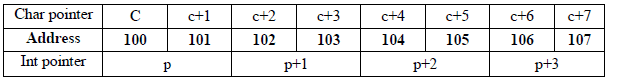Adding 1 to a pointer actually adds the size of pointer’s base type.
Base address : A pointer holds the address of the very first byte of the memory location
where it is pointing to. The address of the first byte is known as BASE ADDRESS.

Dynamic AllocationOperators :
C++ dynamic allocation allocate memory from the free store/heap/pool, the pool of
unallocated heap memory provided to the program. C++ defines two unary operators new and
delete that perform the task of allocating and freeing memory during runtime.

Creating Dynamic Array :
Syntax : pointer-variable = new data-type [size];
e.g. int * array = new int;
Not array will refer to the first element of array, array will refer to the second element.
No initializes can be specified for arrays.
All array sizes must be supplied when new is used for array creation.

Two dimensional array :
int *arr, r, c;
r = 5; c = 5;
arr = new int [r * c];
Now to read the element of array, you can use the following loops :
For (int i = 0; i < r; i++)
{
cout << “ Enter element in row “ << i + 1 << “ : “;
For (int j=0; j < c; j++)
cin >> arr [ i * c + j];
}
Memory released with delete as below: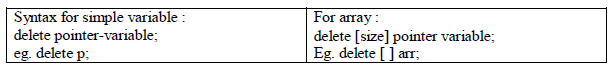Pointers and Arrays :
C++ treats the name of an array as constant pointer which contains base address i.e address of
first location of array. Therefore Pointer variables are efficiently used with arrays for declaration
as well as accessing elements of arrays, because array is continuous block of same memory
locations and therefore pointer arithmetic help to traverse in the array easily.

void main()
{
int *m;
int marks ={ 50,60,70,80,90,80,80,85,75,95};
m = marks; // address of first location of array or we can write it as m=&marks
for(int i=0;i<10;i++)
cout<< *m++;
// or
m = marks; // address of first location of array or we can write it as m=&marks
for(int i=0;i<10;i++)
cout<< *(m+i);
}

Array of Pointers :
To declare an array holding 10 int pointers –
int * ip;
That would be allocated for 10 pointers that can point to integers.
Now each of the pointers, the elements of pointer array, may be initialized. To assign the address of
an integer variable phy to the forth element of the pointer array, we have to write ip = & phy;
Now with *ip, we can find the value of phy. int *ip;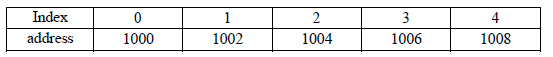int a = 12, b = 23, c = 34, d = 45, e = 56;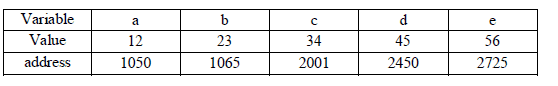ip = &a; ip = &b; ip = &c; ip = &d; ip = &e;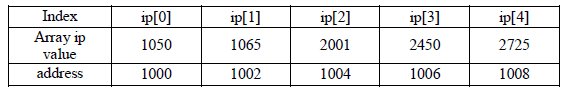ip is now a pointer pointing to its first element of ip. Thus ip is
equal to address of ip, i.e. 1000
*ip (the value of ip) = 1050
* (* ip) = the value of *ip = 12
* * (ip+3) = * * (1006) = * (2450) = 45

Pointers and Strings :
Pointer is very useful to handle the character array also. E.g :
void main()
{ char str[] = “computer”;
char *cp;
cp=str;
cout<<str ; //display string
cout<<cp; // display string
for (cp =str; *cp != ‘’; cp++) // display character by character by character
cout << ”--“<<*cp;
// arithmetic
str++; // not allowed because str is an array and array name is constant pointer
cp++; // allowed because pointer is a variable
cout<<cp;}

Output :
Computer
Computer
--c--o--m--p--u--t--e—r
omputer

An array of char pointers is very useful for storing strings in memory. Char
*subject[] = { “Chemistry”, “Phycics”, “Maths”, “CS”, “English” };
In the above given declaration subject[] is an array of char pointers whose element pointers contain
base addresses of respective names. That is, the element pointer subject stores the base address of
string “Chemistry”, the element pointer subject stores the above address of string “Physics” and so
forth.
An array of pointers makes more efficient use of available memory by consuming lesser number
of bytes to store the string.
An array of pointers makes the manipulation of the strings much easier. One can easily exchange
the positions of strings in the array using pointers without actually touching their
memory locations.

Pointers and CONST :
A constant pointer means that the pointer in consideration will always point to the same
A pointer to a constant refers to a pointer which is pointing to a symbolic constant. Look the
following example :

int m = 20;                                    // integer m declaration
int *p = &m;                                 // pointer p to an integer m
++ (*p);                                         // ok : increments int pointer p
int * const c = &n;                      // a const pointer c to an intger n
++ (* c);                                      // ok : increments int pointer c i.e. its contents
++ c;                                           // wrong : pointer c is const – address can’t be modified
const int cn = 10;                     // a const integer cn
const int *pc = &cn;                 // a pointer to a const int
++ (* pc);                                   // wrong : int * pc is const – contents can’t be modified
++ pc;                                       // ok : increments pointer pc
const int * const cc = *k;        // a const pointer to a const integer
++ (* cc);                                 // wrong : int *cc is const
++ cc;                                      // wrong : pointer cc is const

Pointers and Functions :
A function may be invoked in one of two ways :
1. call by value

2. call by reference

The second method call by reference can be used in two ways :

1. by passing the references

2. by passing the pointers

Reference is an alias name for a variable. For ex : int m = 23;
int &n = m;
int *p;
p = &m;
Then the value of m i.e. 23 is printed in the following ways : cout <<
m; // using variable name
cout << n; // using reference name
cout << *p; // using the pointer

Invoking Function by Passing the References :
When parameters are passed to the functions by reference, then the formal parameters become references (or aliases) to the actual parameters to the calling function.
That means the called function does not create its own copy of original values, rather, it refers to the
original values by different names i.e. their references.

For example the program of swapping two variables with reference method :
#include<iostream.h>
void main()
{
void swap(int &, int &);
int a = 5, b = 6;
cout << “ Value of a :” << a << “ and b :” << b;
swap(a, b);
cout << “ After swapping value of a :” << a << “and b :” << b;
}
void swap(int &m, int &n)
{
int temp; temp = m;
m = n;
n = temp;
}

output :
Value of a : 5 and b : 6
After swapping value of a : 6 and b : 5

Invoking Function by Passing the Pointers:
When the pointers are passed to the function, the addresses of actual arguments in the calling function
are copied into formal arguments of the called function.
That means using the formal arguments (the addresses of original values) in the called function,
we can make changing the actual arguments of the calling function.
For example the program of swapping two variables with Pointers :
#include<iostream.h>
void main()
{
void swap(int *m, int *n);
int a = 5, b = 6;
cout << “ Value of a :” << a << “ and b :” << b;
swap(&a, &b);
cout << “ After swapping value of a :” << a << “and b :” << b;
}
void swap(int *m, int *n)
{
int temp;
temp = *m;
*m = *n;
*n = temp;
}
Input :
Value of a : 5 and b : 6
After swapping value of a : 6 and b : 5

Function returning Pointers :
The way a function can returns an int, an float, it also returns a pointer. The general form of prototype
of a function returning a pointer would be
Type * function-name (argument list);

#include <iostream.h>
int * min(int &, int &); void main()
{
int a, b, *c;
cout << “ Enter a :”; cin >> a;
cout << “ Enter b :”; cint >> b;
c = min(a, b);
cout << “ The minimum no is :” << *c;
}
int *min(int &x, int &y)
{
if (x < y )
return (&x);
else
return (&y)
}

Dynamic structures :
The new operator can be used to create dynamic structures also i.e. the structures for which the
memory is dynamically allocated.
struct-pointer = new struct-type;
student *stu;
stu = new Student;
A dynamic structure can be released using the deallocation operator delete as shown below :
delete stu;

Objects as Function arguments :
Objects are passed to functions in the same way as any other type of variable is passed.
When it is said that objects are passed through the call-by-value, it means that the called function
creates a copy of the passed object.
A called function receiving an object as a parameter creates the copy of the object without invoking
the constructor. However, when the function terminates, it destroys this copy of the
object by invoking its destructor function.
If you want the called function to work with the original object so that there is no need to
create and destroy the copy of it, you may pass the reference of the object. Then the called function
refers to the original object using its reference or alias.
Also the object pointers are declared by placing in front of a object pointer’s name. Classname
* object-pointer;
Eg. Student *stu;

The member of a class is accessed by the arrow operator (->) in object pointer method.

Eg :
#include<iostream.h>
class Point

{
int x, y;
public :
Point()
{x = y = 0;}
void getPoint(int x1, int y1)
{x = x1; y = y1; }
void putPoint()
{ cout << “
Point :
(“ << x
<< “, “
<< y
<< “)”;
}};
void main()
{
Point p1, *p2;
cout << “ Set point at 3, 5 with object”;
p1.getPoint(3,5);
cout << “ The point is :”;
p1.putPoint();
p2 = &p1;
cout << “ Print point using object pointer :”;
p2->putPoint();
cout << “ Set point at 6,7 with object pointer”;
p2->getPoint(6,7);
cout<< “ The point is :”;
p2->putPoint();
cout << “ Print point using object :”;
p1.getPoint();}
If you make an object pointer point to the first object in an array of objects, incrementing the pointer
would make it point to the next object in sequence.
student stud, *sp;
---
sp = stud;               // sp points to the first element (stud)of stud
sp++;                      // sp points to the second element (stud) of stud sp + = 2;
// sp points to the fourth element (stud) of stud sp--;

// sp points to the third element (stud) of stud
You can even make a pointer point to a data member of an object. Two points should be
considered :
1. A Pointer can point to only public members of a class.
2. The data type of the pointer must be the same as that of the data member it points to.

this Pointer :
In class, the member functions are created and placed in the memory space only once. That is
only one copy of functions is used by all objects of the class.
Therefore if only one instance of a member function exists, how does it come to know which object’s
data member is to be manipulated?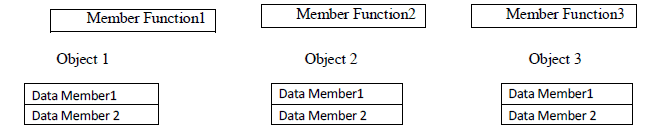For the above figure, if Member Function2 is capable of changing the value of Data Member3
and we want to change the value of Data Member3 of Object3. How would the Member Function2
come to know which Object’s Data Member3 is to be changed?
To overcome this problem this pointer is used.
When a member function is called, it is automatically passed an implicit argument that is a pointer to
the object that invoked the function. This pointer is called This.
That is if ojbect3 is invoking member function2, then an implicit argument is passed to member
function2 that points to object3 i.e. this pointer now points to object3.
The friend functions are not members of a class and, therefore, are not passed a this pointer. The static
member functions do not have a this pointer.

9 docs

,

,

,

,

,

,

,

,

,

,

,

,

,

,

,

,

,

,

,

,

,

,

,

,

,

,

,

,

,

,

;